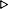ToC DocOverview CGDoc RelNotes Index PermutedIndex
Allegro CLANSI Common Lisp9 Conditions9.2 Dictionary of Conditions

 9.2.10 assert Macro

Syntax:
assert test-form [({place}*) [datum-form {argument-form}*]]nil

Arguments and Values:
test-form - a form; always evaluated.

place - a place; evaluated if an error is signaled.

datum-form - a form that evaluates to a datum. Evaluated each time an error is to be signaled, or not at all if no error is to be signaled.

argument-form - a form that evaluates to an argument. Evaluated each time an error is to be signaled, or not at all if no error is to be signaled.

datum, arguments - designators for a condition of default type error. (These designators are the result of evaluating datum-form and each of the argument-forms.)

Description:
assert assures that test-form evaluates to true. If test-form evaluates to false, assert signals a correctable error (denoted by datum and arguments). Continuing from this error using the continue restart makes it possible for the user to alter the values of the places before assert evaluates test-form again. If the value of test-form is non-nil, assert returns nil.

The places are generalized references to data upon which test-form depends, whose values can be changed by the user in attempting to correct the error. Subforms of each place are only evaluated if an error is signaled, and might be re-evaluated if the error is re-signaled (after continuing without actually fixing the problem). The order of evaluation of the places is not specified; see Section 5.1.1.1 Evaluation of Subforms to Places. If a place form is supplied that produces more values than there are store variables, the extra values are ignored. If the supplied form produces fewer values than there are store variables, the missing values are set to nil.

Examples:
``` (setq x (make-array '(3 5) :initial-element 3))#2A((3 3 3 3 3) (3 3 3 3 3) (3 3 3 3 3))
(setq y (make-array '(3 5) :initial-element 7))#2A((7 7 7 7 7) (7 7 7 7 7) (7 7 7 7 7))
(defun matrix-multiply (a b)
(let ((*print-array* nil))
(assert (and (= (array-rank a) (array-rank b) 2)
(= (array-dimension a 1) (array-dimension b 0)))
(a b)
"Cannot multiply ~S by ~S." a b)
(really-matrix-multiply a b)))MATRIX-MULTIPLY
(matrix-multiply x y)Correctable error in MATRIX-MULTIPLY:Cannot multiply #<ARRAY ...> by #<ARRAY ...>.Restart options:1: You will be prompted for one or more new values.2: Top level.Debug> :continue 1Value for A: xValue for B: (make-array '(5 3) :initial-element 6)#2A((54 54 54 54 54)
(54 54 54 54 54)
(54 54 54 54 54)
(54 54 54 54 54)
(54 54 54 54 54))
```

``` (defun double-safely (x) (assert (numberp x) (x)) (+ x x))
(double-safely 4)8

(double-safely t)Correctable error in DOUBLE-SAFELY: The value of (NUMBERP X) must be non-NIL.Restart options:1: You will be prompted for one or more new values.2: Top level.Debug> :continue 1Value for X: 714
```

Affected By:
*break-on-signals*

The set of active condition handlers.